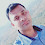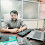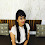# Data Type in Python,What is Data Type in Python

51

The data type is used to provide a pattern, size, and memory allocation of data using variable, constant, and method.

## Python uses data type internally in the program, no need to write a data type declaration in the program because python provides flexibility to the identifier to contain multiple types of data using a single name identifier.

a=10
a= "hello"
a= True
Type of Data type:-
Primitive Type:-  it is the primary data type of Python that is common for all programming languages.
1 int :-       a=10
2 float:-    a=12.34
3 String:-  Collection of Char's
1)  String With Double Quote:-
It used to declare outer String on Python Script.
s= "data"
2)  String With Single Quote:-
It is used to declare inner String on Python Script.
s= 'data'
3)  String With double quote three times
s= """data"""    it support paragraph pattern or multiline statements
4)  String with a single quote three times:-
It is used to provide a multiline comment block.
''' comment '''     multiline comment
Example of String -
a = "<h1 style='color:red'>Welcome</h1>"
print(a)
b = 'hello ahdfbjhdbfhfd'
print(b)
c = """ hello dkjfhdkhfgdhg fjidkjfdkjfgjkdfkdg fhgdhfg hdagfhjdgfdfdfhdfjh
dfhgdhfg hjdg fhjdsg fhgdhfgdhjfghdgfhjdsgfhjdghjfgdhjfgdh """
print(c)
4 boolean:-
it is used to create checkpoint or true|false value in the variable
by default, if statement, loop statement return a boolean value
b = True
b= False
boolean type basically used on a conditional statement, loop statement
Most Important Question of Boolean Data Type:-
s = True
print(s+1)
s1 = False
print(s1+1)
print(s+s1)
Derived Data type:-
this type of data type is specially created for Python Script.
1  LIST:-  it is used to contain a set of elements using an index from 0 to size-1 whose value can be change
2 TUPLE:-  it is used to contain a set of elements using the index value pair whose value can not be changed
3 DICTIONARY:-  it is used to contain a set of elements using key: value pair
4 Set:-  It is used to display elements randomly.
5 DATETIME:-  it is used to contain current data and time
6 Class:-  it is used to create a User-define type
7 Complex:-  it is used to contain a complex number
a = 2+3j    j means iota
........................................................................................................

Predefine function in Python for Data type
type():-   it is used to represent the data type of an identifier in Python. it returns Classname: Datatype under generic pattern.
If we want to display only data type name then python provides __name__ attribute to get the datatype.
a=10
```print(type(a))
a=20.2
print(type(a))
a=2+3j
print(type(a))
a=(10,20)
print(type(a))
a=[10,20]
print(type(a))
a={'a':10,'b':20}
print(type(a))
a=True
print(type(a))
a='hello'
print(type(a))
a="hello"
print(type(a))
a="""hello"""
print(type(a))```
int():-  this method is used to convert numeric String to integer
a= "123"
a = int(a)  #123
float():-  this method is used to convert numeric float String or integer to float
a=12
a = float(12)  #12.0
a= "12.34"
a=float(a)  #12.34
str():-  this method is used to convert int, float type value to String type
a= 12
b=str(a)  #"12"
b = str(12.34)  #"12.34"
ord():-  to convert char to ASCII Code then we use ord().
A ----> 65
a ----> 97
0 ---> 48
9 --->  57
A  to Z      65 to 90
a to z        97 to 122
0 to 9        48  to 57
,,,,,,,,,,,,,,,,,,,,,,,,,,,,,,,,,,,,,,,,,,,,,,,,,,,,,,,,,,,,,,,,,,,,,,,,,,,,,,,,,,,,,,,,,,,,,,,,,,,,,,,,,,,,,,,,,,,,,,,,,,,,,,,,,,,,,,,,,,,,,,,,,,,,,,

Chr():-    It is used to convert ASCII Code to char
a = 90
print(chr(a))     Z
Q WAP to convert temperature from Celsius to Fahrenheit?
Q WAP to perform multiplication of a complex number using complex data type?
Q WAP to evaluate the middle number in three-digit numbers based on order?

a= 132   #2
a=456  #5
a = 546  #5
Q WAP to print data in the single quote and double quote
`"Welcome in SCS" 'Welcome in Python' '\n hello \n"`
Tags

1.Program of multiplication of complex numbers using complex data type-->

x=complex(3+5j)
print(x)
y=complex(2+3j)
print(y)
multiply=x*y
print("multiplication of complex numbers=",multiply)

2.Program to convert temperature from Celsius to Fahrenheit-->

c=float(input("enter the value of c"))
f=(9*c+160)/5
print("temprature in fahrenheit=",f)

3.Program to evaluate the middle number in three-digit numbers based on order-->

num=int(input("enter the three digit number"))
a=num%100
a=a//10
print("mid number is=",a)

4.wap tempuratre celsius to farahenite
temp=float(input("enter temp in celsius"))
tf=((temp*(9/5)+32)
print(tf)

5.wap tempurature farahnite to celsius
tempf=float(input("enter temp in farhenite")
tc=((temp-32)*(5/9))
print(tc)

6.wap to perform multipication of complex number using complex data type
comp1=complex(input("enter 1st complex number"))
comp2=complex(input("enter 2nd complex number"))
c=(com1*comp2)
print(c)

7.Lokesh Rathore
Multiplication of Complex Number using Complex Data Type

Solution:-
a=complex(2+5j)
b=complex(4+8j)
c=a*b
print("The Multiplication of Complex Number is :- ", c)

8.Lokesh Rathore

Print data in the single quote and double quote ?
Solution:-
print("'Hello Lokesh !!!!'")
'''To Print the String in single Quote, Write the sentence in Double Quote with Single Quote.'''
print('"Hello Lokesh !!!!"')
'''To Print the string in Double Quote , Write the sentence in Single Quote with Double Quote.'''

9.deependra singh jadaunNovember 3, 2020 at 8:31 PM

f=((9/5)*c)+(32)
print(f)

10.wap to multiply complex no.
a=2+3j
b=3+5j
print(a*b)

11.program to find mid no.
number=int(input("enter the three digit number"))
a=number%100
a=a//10
print("middle number is=",a)

12.program to print any data in single quote and double quote-:
d=input("enter any data")
print("'{}'" .format(d))
print('"{}"'.format(d))

13.# PYTHON (6 To 7 PM BATCH)
# Multiplication of a complex number using complex data type.

a = complex(input("Enter the First Complex (a+bj) No. :\t"))
b = complex(input("Enter the Second Complex (a+bj) No.:\t"))
print("\nResult =\t",a*b)

14.#PYTHON( 6 To 7 PM BATCH)
#To Find the middle number in three-digit numbers based on order .

a=int(input("Input First number: "))
b=int(input("Input Second number: "))
c=int(input("Input Tird number: "))
if(b<a and a<c):
print("Middle Number:\t",a)
elif(a<b and b<c):
print("Middle Number:\t",b)
elif(a<c and c<b):
print("Middle Number:\t",c)
pass

15.Parag Jaiswal

#WAP to convert temperature from Celsius to Fahrenheit?

cel = int(input("Enter temperature = "))

fah = (cel * 1.8) +32

print("Temperature in fahrenheit is = ", fah)

16.Parag Jaiswal
#WAP to perform multiplication of a complex number using complex data type?

a =complex(3+5j)
b =complex(2+4j)

print(a*b)

17.Parag Jaiswal
3 WAP to evaluate the middle number in three-digit numbers based on order?

x = int(input("Enter 3 digit number = "))
a = x % 100
b = a // 10
print("Middle number is = ",b)

18.Parag Jaiswal
#WAP to print data in the single quote and double quote

print('"Stay home, stay safe"')
print("'Use hand sanitizer & wear mask '")

19.multiplication of a complex number:-
a=2+5j
b=4+6j
print("a=",a)
print("b=",b)
c=(a*b)
print("multiplication of a complex number:",c)

20.t_c = int(input("Enter Temperature Which you want to Convert into Fahrenheit = "))

t_f = ((t_c)*9/5+32)

print(t_f)

21.print("print Message in within single quotes")
print("'Hello Python'")

22.print("Print Message in within Double Quotes")
print('"Hello Python"')

23.Reverse five digit number without using loop?
num = 45798
a = num%10 #5
num = num//10 #1234
b = num%10 #4
num= num//10 #123
c = num%10 #3
num= num//10 #12
d = num%10
num= num//10 #1
e = num%10
num1 = a*10000+b*1000+c*100+d*10+e*1
print(num1)

24.#NIKHIL SINGH CHOUHAN
'''WAP to evaluate the middle number
in three-digit numbers based on order?'''

i=int(input("enter number"))
a=i%100
b=a//10
print("middle number is=",b)

25.##Program for celcius to Fahrenheit..#Jeet7

temperature=float(input("enter the value of c"))
s=(9*temperature+160)/5
print("temprature in fahrenheit=",s)

26.#convert temperature from Celsius to Fahrenheit?
celsius = float(input("Enter the temperatire in Cilsius : "))
fahrenheit = (celsius * 1.8) + 32
print(" %.2f C = %.2f F " %(celsius,fahrenheit))

27.c=99
f=1.8*c+32
print(f)

28.#WAP TO CONVERT TEMP.FROM CELSIUS TO FAHRENHEIT?
C=99
F=1.8*C+32
print(F)

29.#Wap to multiplication of complex no using complex data type?
p,q=2+3j,3+4j
r=p*q
print(r)

30.#Wap to print data in the single quote & double quote?
print("display'hello python'on monitor")
print('display"hello python"on monitor')

31.#Surendra Singh Rajput
c=float(input("enter the value"))
Value =(9*c+160)/5
print("temprature =",Value)

32.#wap to find mid num in e digit number
#jeet

Num= int(input("Enter 3 digit number = "))
x = Num % 100
x= x// 10
print("Middle number is = ",x)

33.convert celcius to Fahrenheit

c=float(input("enter temperature in celcius"))
f=(c*9/5)+ 32
print("the temperature in fahrenheit is", f)

34.multiplication of complex no.

a=complex(2+4j)
b=complex(4+5j)
c=a*b
print(c)

35.multiplication of a complex no.

a=complex(2+4j)
b=complex(4+5j)
c=a*b
print(c)

36.print("'welcome in python'")

print('"welcome in SCS"')

37.Num= int(input("Enter 3 digit number "))
a= Num % 100
a= a// 10
print("number is = ",a)

38.a=complex(input("enter First complex number"))
b=complex(input("enter Second Complex number"))
c=a*b
print (c)

39.SONAM SINGH
WAP to convert the multiplication of complex number
num1=complex(input("enter the first complex number"))
num2=complex(input("enter the second complex number"))
complexformula=num1*num2
print(complexformula)

40.#WAP to perform addition and mutiplication complex number without using complex data type
r1=34
r2=25
i1=12
i2=15
r=r1*r2-i1*i2
i=r1*i2+r2*i1
print("{0}+{1}i".format(r,i))
r=r1+r2
i=i1+i2
print("{0}+{1}i".format(r,i))

41.#WAP to convert temperature from Celsius to Fahrenheit and vice versa
print("celsius to fahrenheit")
celsius=float(input("enter the temperature in celsius"))
fahrenheit=(celsius*9/5)+32
print(fahrenheit)

print("fahrenheit to celsius")
fahrenheit=float(input("enter the temperature in fahrenheit"))
celsius=(fahrenheit-32)*5/9
print(celsius)

42.#WAP to perform multiplication of a complex number using complex data type
x=complex(input("enter first number"))
y=complex(input("enter second number"))

multiplication=x*y
print("the multiplication of complex number is", multiplication)

43.#celcius to fahrenhet
# f=(c*9/5)+(32)

c=float(input("enter the temp. in c"))
f=(c*9/5)+(32)
print("the temp.in fahrenhiet to celcius is",c,f)

44.num=int(input("Enter Any Number"))
a=num%100
a=a//10
print("Middle Number is ",a)

45.# wap to print data in the single quote and double quote?
print("'how are you'")
print('" i am fine"')

46.# wap to convert temperature from celsius to fahrenheit?
c=float(input("enter the value of c="))
f=(9*c+160)//5
print("temperature in fahrenheit=s",f)

47.a=3+4j
print(a)
b=5+6j
print(b)
c=a*b
print("multiplication of complex number =",c)

48.# wap to display the middle number is a three - digit number?
num=int(input("enter three digit number"))
r1=num%10
num1=num//10
r2=num1%10
print(r2)

49.multiplication of a complex number using complex data type?

x= complex(2,3)
y=complex(2,3)
z=x*y
print("Multiplication of complex No",z)

50.Devendra Patil

x= complex(2,3)
y=complex(2,3)
z=x*y
print(z)

51.a="'hello world'"
print(a)

b='"hello mangal"'

output - 'hello world'
"hello mangal"